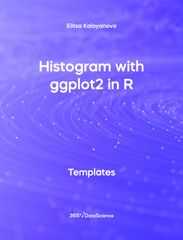# Templates

Boost your programming skills with free Python, SQL, R, and Excel templates and create top-notch projects for your portfolio.

Save hours of browsing through the Internet and accelerate your workflow with code-ready templates for your programming projects. Personally crafted by 365 Data Science instructors, these templates bring a surprising efficiency level to your work process.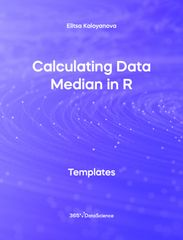Templates r

## Calculating Data Median in R Template

The following Calculating Data Median in R template shows the mean, also known as the simple average. We can find the mean of a data set by adding up all of its components and then dividing them by the number of components contained in the data set. Some other related topics you might be interested in exploring are Calculating Data Variance in R, Calculating Data Median in R, Calculating Standard Deviation of Data in R and Exploring Data Skewness in R. You can now download the R template for free. Calculating Data Median in R is among the topics covered in detail in the 365 Data Science program.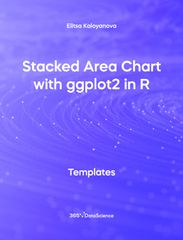Templates r

## Stacked Area Chart with ggplot2 in R Template

The following Stacked Area Chart with ggplot2 in R template shows the popularity of different engine types in automobiles across several decades. An area chart is used when we are interested in tracking and comparing the volume of a few variables over time. In a stacked area chart each category is ‘stacked’ or ‘placed’ on top of the previous and there is no overlap among categories. Some other related topics you might be interested in exploring are Line Chart with ggplot2 in R, Bar Chart with ggplot2 in R, Pie Chart with ggplot2 in R and Scatter Plot with ggpot2 in R. You can now download the R template for free. Stacked Area Chart with ggplot2 in R is among the topics covered in detail in the 365 Data Science program.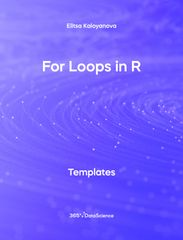Templates r

## For Loops in R Template

The following For Loops in R shows how to calculate the sum of the numbers from 1 to 10 using a for loop. For loops are one of the most commonly used tools by any programmer and they are used when we want to repeat an action in the loop for a certain number of times. Some other related topics you might be interested in exploring are While Loops in R and If Else Else If statements .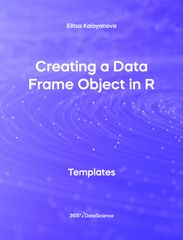Templates r

## Creating a Data Frame Object in R Template

The following Creating a Data Frame Object in R template shows you how to create a data frame in R from scratch and populate with different values. The example shows a car data frame, containing 5 columns, 3 of which are numeric and 2 of which are strings. You can use this template to create and fill out your own data frame. Some other related topics you might be interested to explore are Calculating Data Variance in R, Calculating Data Mean in R, Calculating Standard Deviation of Data in R, Exploring Data Skewness in R. You can now download the R template for free. Creating a Data Frame Object in R is among the topics covered in detail in the 365 Data Science program.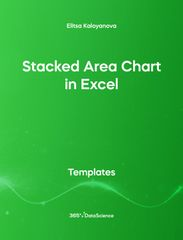Templates excel

## Stacked Area Chart in Excel Template

This Stacked Area Chart in Excel template displays the use of different fuel types from the year 1975 to 2016 for used cars. The chart is created with the stacked area chart in Excel. For completion a 100% stacked version of the chart is shown. Some other related topics you might be interested to explore are Pie Chart in Excel, Line Chart in Excel, and Scatter Plot in Excel. You can now download the Excel template for free. Stacked Area Chart is among the topics covered in detail in the 365 Data Science program.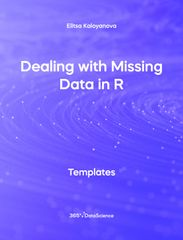Templates r

## Dealing with Missing Data in R Template

This Dealing with Missing Data in R template shows you how to check for missing values in a dataset and count them. It also provides two ways of dealing with missing values in a dataset - either by substituting the missing values with the average, or by removing the entries which have missing values in them. Some other related topics you might be interested to explore are correlation between two variables in R, export data as csv in R, calculating data median in R, calculating data mean in R, and calculating standard deviation of data in R. You can now download the R template for free. Dealing with missing data in R is among the topics covered in detail in the 365 Data Science e-learning program.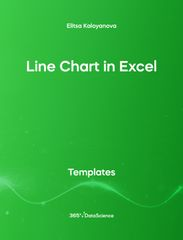Templates excel

## Line Chart in Excel Template

A line chart is often used when we want to chronologically track the changes in value of a variable over a period of time and identify existing patterns and trends. Therefore, the line chart is often applied in financial statements, weather forecasts, stock market analysis and experiment statistics reports. This free .xlsx template displays the S&P 500 and Footsie indices for the second half of the economically devastating 2008 on a line chart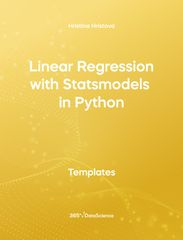Templates python

## Linear Regression with statsmodels in Python Template

The following Linear Regression with Statsmodels in Python free .ipynb template shows how to solve a simple linear regression problem using the Ordinary Least Squares statsmodels library. We are going to examine the causal relationship between the independent variable in the dataset - SAT score of a student, and the dependent variable -the GPA score. This database is read with the help of the pandas library. Download and unzip the .zip file in a new folder. Inside the folder you will find a .csv and a .ipynb file. The first one contains the database and the second one contains the Python code. Open the .ipynb file using Jupyter notebook.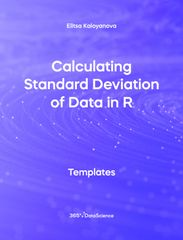Templates r

## Calculating Standard Deviation of Data in R Template

This free .r template will show you how to calculate the standard deviation of data using the R programming language. The standard deviation is obtained by taking the square root of the variance of the data. It is used in many statistical analyses, whenever we have numerical data and is a measure of data dispersion. The standard deviation shows how closely data sits around the mean – a small value shows data, which is densely populated around the mean, a large standard deviation signifies data which is more dispersed.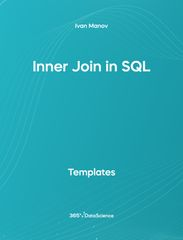Templates SQL

## Inner Join in SQL Template

Joins are the SQL tools that allow us to work with data from multiple tables simultaneously relying on the logical relationship between their objects. The INNER JOIN clause, in particular, creates a new instance of a table that combines rows with matching values from two tables. Thus, null values, or values appearing only in one of the tables, will not be extracted. In this free sql template you will be applying the SQL Inner Join clause on a set of business department tables.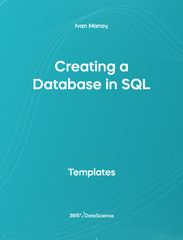Templates SQL

## Creating a database in SQL Template

Developed in the 1970s by a group of IBM researchers, SQL continues to be the most popular programming language for relational database management and is used by companies like Facebook to store mounts of user data. None of this would be possible without the foundation of the SQL language- the database. That is the place where information is organized into tables and can be accessed, manipulated, and retrieved in any desired way. Consider this as the very first step in your SQL journey as this free sql template will show you how to make a SQL database in MYSQL.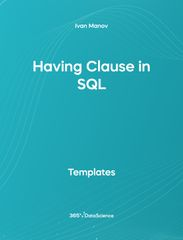Templates SQL

## Having Clause in SQL Template

SQL is specifically designed to help you navigate through large amounts of structured data, modifying, retrieving, and creating new tables out of it. The having clause works in conjunction with common aggregate functions like min, max, ave, count and sum, and allows you to filter and return group results that meet the specified conditions. In this free sql template, you will apply the having clause to display salaries that are higher than a certain value in ascending order.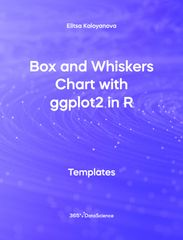Templates r

## Box and Whiskers Chart with ggplot2 in R

A box and whiskers chart graphically represents the distribution of data through their quartiles. It is often used in financial settings when analyzing the market volatility and can reveal the skewness of data or potential outliers. Тhis free .r template goes over the Titanic’s data set using the ggplot2 library in R, revealing interesting insights about e the survival rate based on age and sex. By following the outlined steps in this R template, you will learn how to convey the information professionally using the ggplot2 functionalities.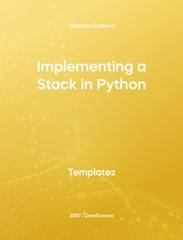Templates python

## Implementing a Stack in Python

Inevitably, all of us have experienced the stack structure in our daily lives- whether it is shuffling a deck of cards, using the undo button in Word, or browsing back and forth between webpages. In the following free .ipynb notebook, you will find an implementation of a Stack class from scratch – showing you how to check the stack’s status, append(push) as well as remove(pop) items from the stack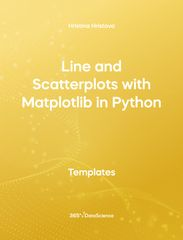Templates python

## Line and Scatterplots with Matplotlib in Python

The following notebook will guide you through the process of creating a line plot and a scatter plot using the matplotlib library in Python. The data that you are going to be working with in this free .ipynb template is the number of daily views on a video for a period of 30 days. You will learn how to customize the style of your plot by changing the figure sizes, and the line’s style and colors. You will also learn how to put markers on the plot, give name to the axes and put a title on the figure.Templates python

## Incorporating URL parameters into a GET request in Python Template

A GET request is used when we want to obtain a certain document from a server- like a web page or API output. However, we can also add different parameters to the request to obtain a more specific result – either by modifying or adding additional information. In this free .ipynb template, we will show you how to incorporate such parameters into the URL by using the "requests" Python package.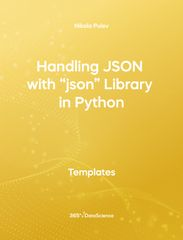Templates python

## Handling JSON with “json” library in Python Template

This is a free .ipynb template demonstrating how to handle JSON files using Python and the 'json' library. JSON is a standard data exchange format, frequently encountered on the web, mainly as the output of APIs. It uses 2 main datatypes common to many programming languages - lists and dictionaries, which are going to be covered in the template.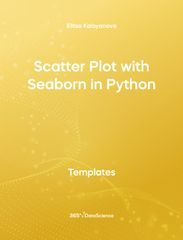Templates python

## Scatter Plot with Seaborn in Python Template

The seaborn library has been one of the most popular Python libraires in recent years. Compared to matplotlib, seaborn has simpler and more intuitive syntax, and wider visual-enhancing features. This free .ipynb Scatter Plot with Seaborn in Python template shows the relationship between the price and area of houses, based on real estate data. It's easy and intuitive to build and customize a scatter plot with the help of seaborn.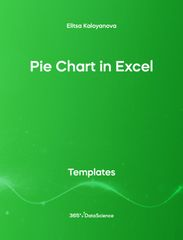Templates excel

## Pie Chart in Excel Template

Pie charts are one of the most popular data visualization tools since they express the part-to whole relationship of a dataset in a very intuitive manner. As such they are best used when we want to communicate for example the revenue of each product and its relationship to the whole. The following free. xlsx template shows an Excel pie chart, displaying the number of cars using a particular type of fuel.